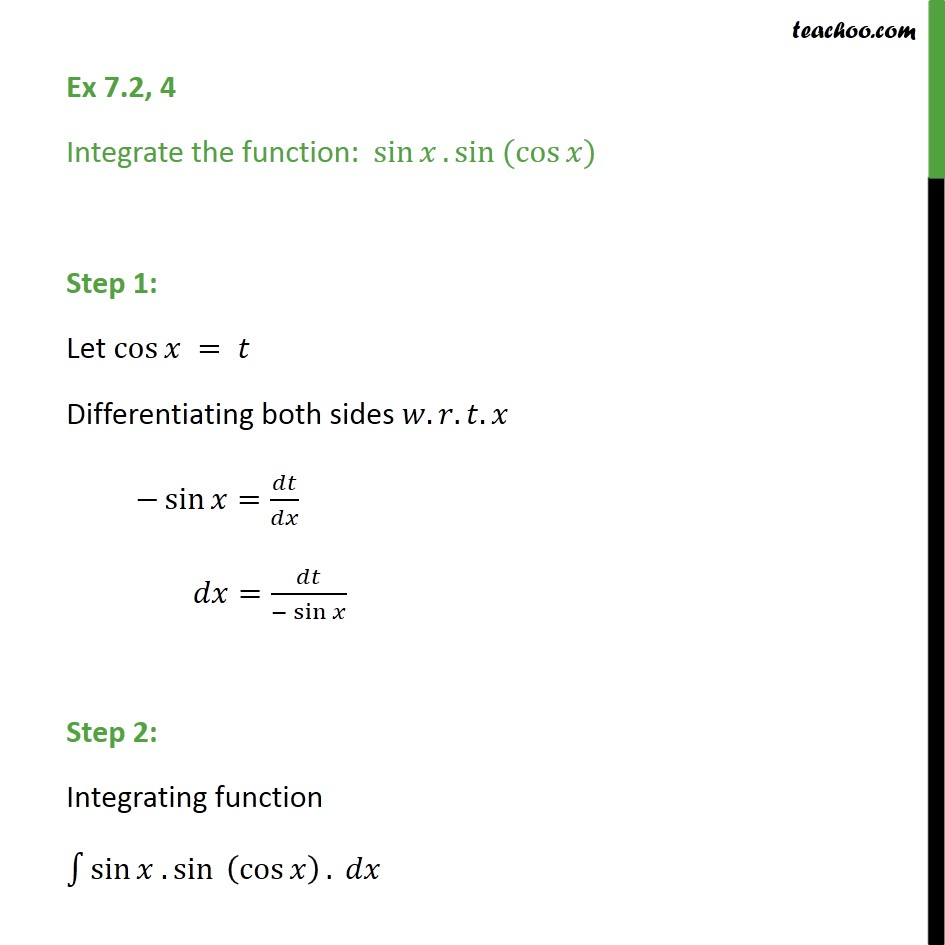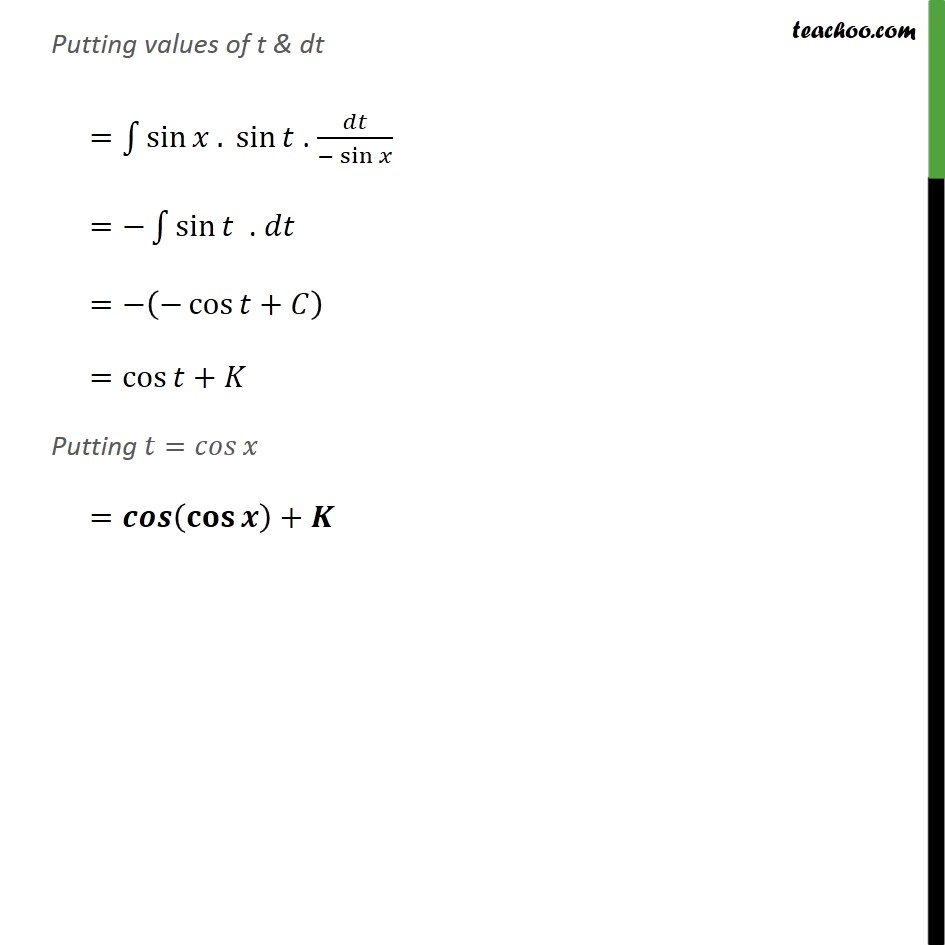1. Chapter 7 Class 12 Integrals (Term 2)
2. Serial order wise
3. Ex 7.2

Transcript

Ex 7.2, 4 Integrate the function: sin . sin (cos ) Step 1: Let cos = Differentiating both sides . . . sin = / = /( sin ) Step 2: Integrating function 1 sin . sin (cos ) . Putting values of t & dt = 1 sin . sin . /( sin ) = 1 sin . = ( cos + ) =cos + Putting = = ( )+

Ex 7.2